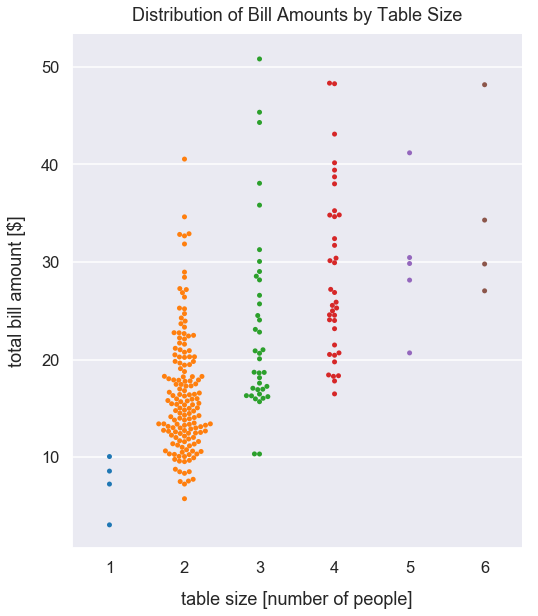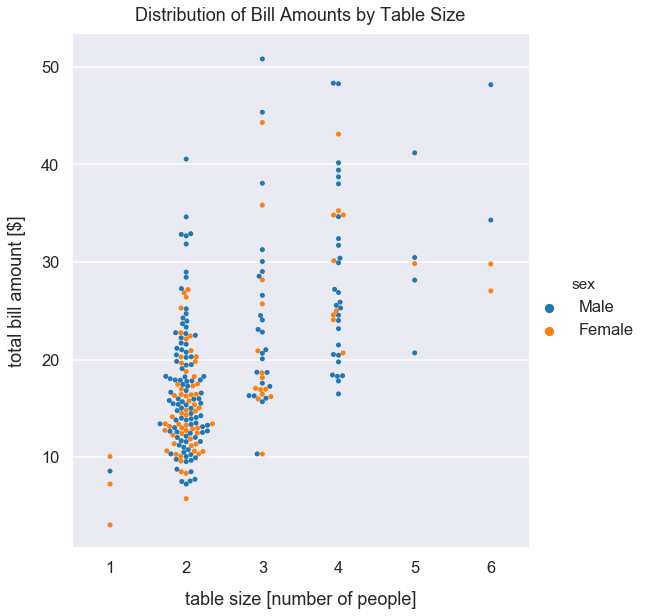Data Visualizations Best Practices Tutorial

# When to Use Categorical Scatterplots

Often times in businesses we want to visualize the distribution from several categorical variables provided in a dataset.

To display the distribution of a category of data, typically people use a box plot or histogram. However, sometimes those visualizations may be improperly used. If you have a small number of data points for one category - often 5 - 80 points, I'd recommend you start with a categorical scatter plot for comparison.

Below, I'll illustrate a few examples.

#### Import Modules¶

In :
import pandas as pd
import seaborn as sns
import matplotlib.pyplot as plt
% matplotlib inline


#### Set Global Styles for Visualizations¶

In :
sns.set_context('talk')
sns.set_style("darkgrid")


### Example: Tips Dataset¶

In the examples below, I utilize the dataset tips provided in the Seaborn visualization library.

Each row in this dataset is a record of a meal at a restaurant. For each meal, the restuarant recorded values for the total bill amount in U.S. dollars, tip amount, gender/sex of the waiter, day, meal and table size.

#### Load flights Dataset¶

In :
df_tips = sns.load_dataset("tips")


#### Preview the First 5 Rows of Data¶

In :
df_tips.head(8)

Out:
total_bill tip sex smoker day time size
0 16.99 1.01 Female No Sun Dinner 2
1 10.34 1.66 Male No Sun Dinner 3
2 21.01 3.50 Male No Sun Dinner 3
3 23.68 3.31 Male No Sun Dinner 2
4 24.59 3.61 Female No Sun Dinner 4
5 25.29 4.71 Male No Sun Dinner 4
6 8.77 2.00 Male No Sun Dinner 2
7 26.88 3.12 Male No Sun Dinner 4

#### Visualize Total Bill Amount by Table Size¶

This categorical scatter plot below, more specifically called a swarm plot, helps illustrate the count of records for each table size and the distribution of bill amounts by table size.

A swarm plot is ideal here because we have so few records of meals with several table sizes such as 1, 5, and 6.

Alternatively, if we tried to use a histogram or box plot to illustrate these few records for category, we'd get a false representation of the bill amounts by table size. The reason for that is that it's bad practice to compare just 4 records for a table size of 1 to 156 record for a table size of 2. For the 4 records of table size of 1 person, those could be a false representation since they may have all made very very small purchases and therefore small total_bill amounts in our dataset.

In :
sns.catplot(x="size", y="total_bill", kind="swarm", data=df_tips, height=8.5, aspect=.9)
plt.xlabel("table size [number of people]", labelpad=15)
plt.ylabel("total bill amount [$]", labelpad=15) plt.title("Distribution of Bill Amounts by Table Size", y=1.013);#### Interpretation of Distribution of Bill Amounts by Table Size¶ There's few records of bill amounts for table sizes of 1, 5, and 6. The restaurant mostly gets table sizes of 2 and 3. Generally, as the table size increases, total bill amount increases. For a table size of 2, most bill amounts are between 10 and 20 U.S. dollars. #### Visualize Total Bill Amount by Table Size and Gender/Sex¶ To improve on the visualization from above, I'm curious if there's any patterns of bill amounts for tables by waiter or waitresses' gender. In : sns.catplot(x="size", y="total_bill", kind="swarm", hue='sex', data=df_tips, height=8.5, aspect=.9) plt.xlabel("table size [number of people]", labelpad=15) plt.ylabel("total bill amount [$]", labelpad=15)
plt.title("Distribution of Bill Amounts by Table Size", y=1.013);#### Interpration of Visualization of Total Bill Amount by Table Size and Gender/Sex¶

There's no clear pattern of bill amounts by table size and gender.

At a glance, it's likely males have served more tables than females. Males often hold the top few spots for highest bill amount by table size too.